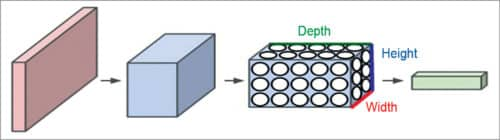Convolutional neural networks (CNNs or ConvNets) used in machine learning are similar to neural networks. These are made up of neurons that have learnable weights and biases. Each neuron receives some inputs, performs a dot product and optionally follows it with a non-linearity. The whole network expresses a single differentiable score function from the raw image pixels on one end to class scores at the other, with a loss function (such as support vector machine/softmax) on the last (fully-connected) layer. Softmax function is just a generalisation of logistic function. It is used as a cost function for probabilistic multi-class classification, and by itself it is not a classifier.

CNNs have revolutionised the pattern-recognition computation process. Prior to the widespread adoption of CNNs, most pattern-recognition tasks involved hand-crafted features extraction followed by classification. With CNNs, features are now learned automatically from training examples. The CNN approach is especially powerful when applied to image recognition because convolution operation captures the 2D nature of images. By using the convolution kernels to scan an entire image, relatively few parameters need to be learned compared to the total number of operations.

## CNN architecture

Unlike a regular neural network, the layers in a CNN have neurons arranged in three dimensions: width, height and depth. Here, ‘depth’ refers to the third dimension of an activation volume, not to the depth of a full neural network, which can refer to the total number of layers in a network.

For example, input images in CIFAR-10 are an input volume of activations, and the volume has dimensions 32x32x3 (width, height and depth, respectively). Neurons in a layer will only be connected to a small region of the layer before it, instead of all of the neurons in a fully-connected manner. Moreover, the final output layer for CIFAR-10 would have dimensions 1x1x10, because by the end of the ConvNet architecture the full image will reduce into a single vector of class scores, arranged along the depth dimension.Fig. 1: Illustration of a ConvNet

Every layer of a CNN transforms the 3D input volume to a 3D output volume of neuron activations. In the example shown in Fig. 1, the red input layer holds the image, so its width and height would be image dimensions, and the depth would be 3 (red, green, blue channels).

Typically, CNN architectures comprise three types of layers stacked together: convolutional layer, pooling layer and fully-connected (FC) layer.

A simple ConvNet for CIFAR-10 classification has the architecture

Input->Conv->Relu->Pool->FC as detailed below:

1. Input [32x32x3] holds the raw pixel values of the image; in this case, an image of width 32 and height 32, with three colour channels R, G and B

2. Conv layer computes the output of neurons that are connected to local regions in the input, each computing a dot product between their weights and a small region they are connected to in the input volume. This may result in a volume such as [32x32x12] if twelve filters are used

3. Relu layer applies an element-wise activation function, such as the max(0,x) thresholding at zero. This leaves the size of the volume unchanged ([32x32x12]).

4. Pool layer performs a down-sampling operation along the spatial dimensions (width, height), resulting in a volume such as [16x16x12]

5. Fully-connected (FC) layer computes class scores, resulting in a volume of size [1x1x10], where each of the ten numbers correspond to a class score, such as among the ten categories of CIFAR-10. Each neuron in this layer is connected to all the numbers in the previous volume.

Thus, CNNs transform the original image layer by layer from the original pixel values to the final class scores. Note that some layers contain parameters, while others don’t. In particular, Conv/FC layers perform transformations that are a function of not only activations in the input volume but also of neuron parameters (weights and biases). On the other hand, Relu/Pool layers implement a fixed function. Parameters in Conv/FC layers are trained with gradient descent so that class scores computed by the CNN are consistent with labels in the training set for each image.

## Architecture for vehicle control

In end-to-end learning system for self-driving cars, weights of the network are trained to minimise the mean-squared error between the steering command output by the network and the command of either the human driver or the adjusted steering command for off-centre and rotated images. Fig. 2 shows the network architecture for vehicle control, which consists of nine layers, including a normalisation layer, five convolutional layers and three fully connected layers.Fig. 2: CNN architecture for vehicle control

The input image is split into YUV planes and passed to the network. The network has about 27 million connections and 250,000 parameters. The first layer of the network performs image normalisation; the normaliser is hard-coded and not adjusted in the learning process. Performing normalisation in the network allows the normalisation scheme to be altered with the network architecture, and accelerated via GPU processing.